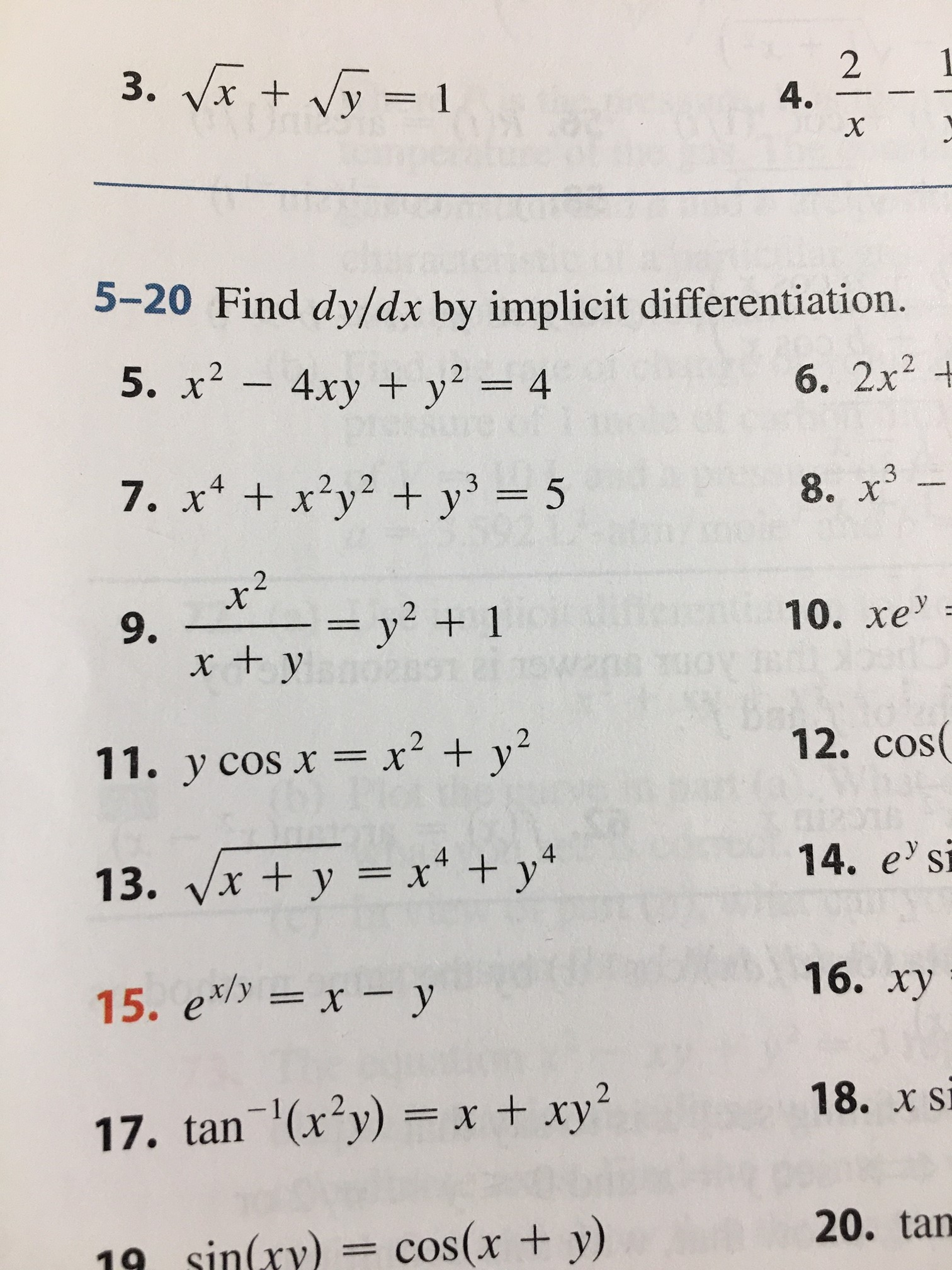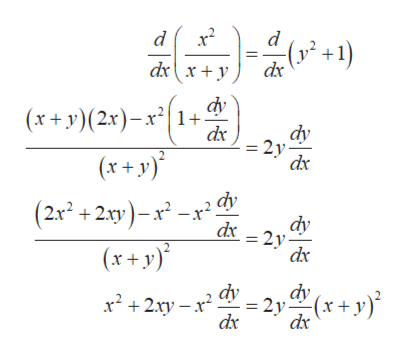# 3. Vxy124.5-20 Find dy/dx by implicit differentiation.5. x24xy y = 46. 2х27. xxy2 y3 52,,28. х329.x + yoen= y2 + 110. хе12. cos(11. y cos x x2 + y2414. e' si13. Vx+ y = x4 + y16. ху15. eyxy18. x s17. tan (xy)x + xy220. tan19 sin(xy) = cos(x + y)

Question
3 views

#9 on the attached picture please.  Find by implicit differentiation.help_outlineImage Transcriptionclose3. Vxy1 2 4. 5-20 Find dy/dx by implicit differentiation. 5. x24xy y = 4 6. 2х2 7. xxy2 y3 5 2,,2 8. х3 2 9. x + y oen = y2 + 1 10. хе 12. cos( 11. y cos x x2 + y2 4 14. e' si 13. Vx+ y = x4 + y 16. ху 15. eyxy 18. x s 17. tan (xy)x + xy2 20. tan 19 sin(xy) = cos(x + y) fullscreen
check_circle

Step 1

Consider given equation:

Step 2

Now, differentiate both sides wi...help_outlineImage Transcriptionclosed d +1) dx dxx+y фy (x+y)(2x)-x1+ dy 2y dx dx (x+y) dy (2x +2ку) -к* - dy 2у dx dx (x+y) dy - 2y (x+y) fullscreen

### Want to see the full answer?

See Solution

#### Want to see this answer and more?

Solutions are written by subject experts who are available 24/7. Questions are typically answered within 1 hour.*

See Solution
*Response times may vary by subject and question.
Tagged in

### Derivative